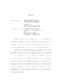## MODEL THEORY AND COMPLEXITY THEORY##### Files
umi-umd-4624.pdf(584.38 KB)
Descriptive complexity theory is a branch of complexity theory that views the hardness of a problem in terms of the complexity of expressing it in some logical formalism; among the resources considered are the number of object variables, quantifier depth, type, and alternation, sentences length (finite/infinite), etc. In this field we have studied two problems: (i) expressibility in $\exists SO$ and (ii) the descriptive complexity of finite abelian groups. Inspired by Fagin's result that $NP=\exists SO$, we have developed a partial framework to investigate expressibility inside $\exists SO$ so as to have a finer look into $NP$. The framework uses combinatorics derived from second-order Ehrenfeucht-Fra\"{\i}ss\'{e} games and the notion of game types. Among the results obtained is that for any $k$, divisibility by $k$ is not expressible by an $\exists SO$ sentence where (1) each second-order variable has arity at most $2$, (2) the first-order part has at most $2$ first-order variables, and (3) the first-order part has quantifier depth at most $3$. In the second project we have investigated the descriptive complexity of finite abelian groups. Using Ehrenfeucht-Fra\"{\i}ss\'e games we find upper and lower bounds on quantifier depth, quantifier alternations, and number of variables of a first-order sentence that distinguishes two finite abelian groups. Our main results are the following. Let $G_1$ and $G_2$ be a pair of non-isomorphic finite abelian groups, and let $m$ be a number that divides one of the two groups' orders. Then the following hold: (1) there exists a first-order sentence $\varphi$ that distinguishes $G_1$ and $G_2$ such that $\varphi$ is existential, has quantifier depth $O(\log{m})$, and has at most 5 variables and (2) if $\varphi$ is a sentence that distinguishes $G_1$ and $G_2$ then $\varphi$ must have quantifier depth $\Omega(\log m)$. In infinitary model theory we have studied abstract elementary classes. We have defined Galois types over arbitrary subsets of the monster (large enough homogeneous model), have defined a simple notion of splitting, and have proved some properties of this notion such as invariance under isomorphism, monotonicity, reflexivity, existence of non-splitting extensions.